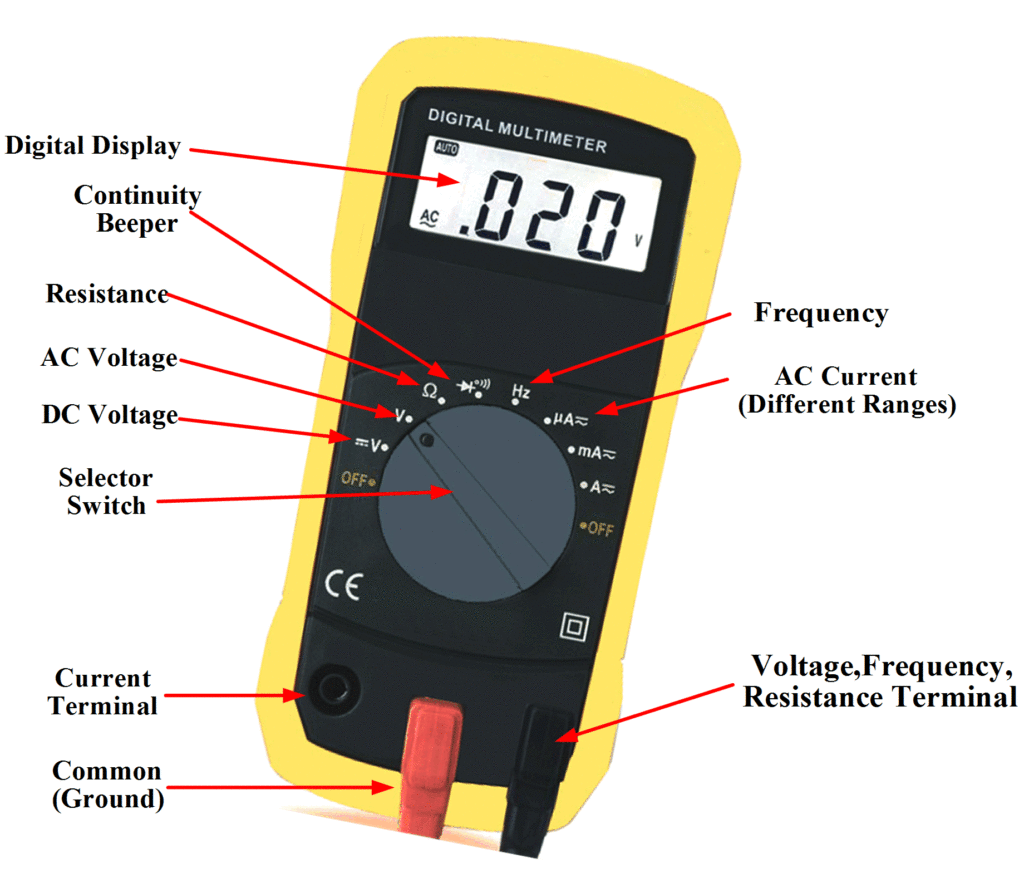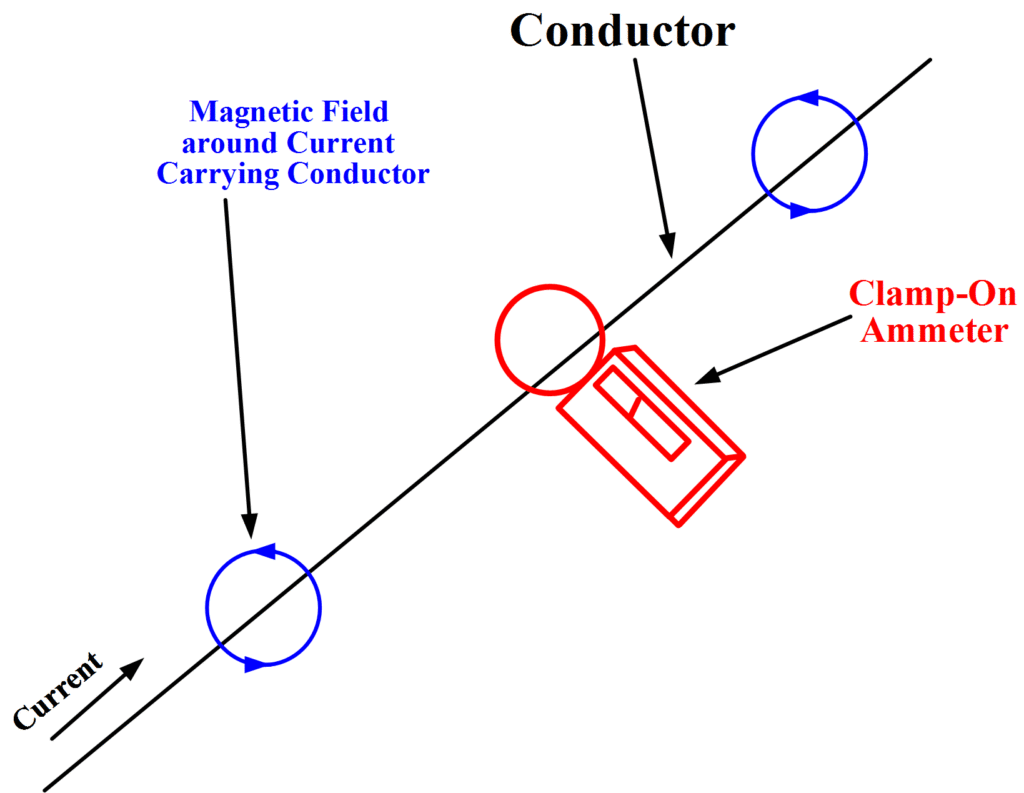# Digital Multimeter Working Principle

Want create site? Find Free WordPress Themes and plugins.

MultimeterA multimeter, shown in Figure 1, is a device used to measure two or more electrical quantities. A multimeter can be used to measure electrical functions such as voltage, current, resistance, continuity and some are able to measure electrical frequency.Fig.1: Digital Multimeter

There are two main types of multimeters.  One of the first and oldest multimeters is the analog meter, (Figure 2) and the other, now more widely used meter is the digital multimeter (Figure 1).Fig.2: Analog Multimeter

## Analog Multimeter

Analog meters are a multifunctional multimeter that operates based on electrical mechanical movement. Analog meters use a printed linear or nonlinear background and a mechanical pointer. The pointer moves as a result of the flow of current through a built-in coil, the presence of electrical pressure, or the internal power source that is needed for resistance measurements. The advantage of an analog meter is relatively small; however, it allows you to see small changes in current flow and a change in voltage in real time. Analog meters require great mathematical skills because you are required to make quick calculations based on the printed scale. Time used while calculating mathematical solutions while taking a reading with an analog meter could be better used to resolve other problems.

## Digital Multimeter

A digital multimeter (DMM) is a multifunctional meter that displays its electrical quantitative values on an LCD screen. A digital multimeter much like an analog meter, it is able to read voltage, current, and resistance. What makes a digital multimeter differ from the analog meter is its ability to display measured electrical values quickly without any computations. Because of its design, a processor can be built into the meter which allows the user to take measurements of frequency, the inductance of a coil, capacitance of a capacitor, and a host of other high functional electrical measurements. There two types of digital multimeters (DMM):  scalable digital multimeter and auto-ranging digital multimeter as shown in Figure 1. When working with the scalable digital multimeter you need to have an idea of the value of voltage, current, or resistance that you are attempting to measure.  Failure to observe these values will result in inaccurate readings and possible damage to the meter. The auto-ranging digital multimeter is more widely used due to its ease, high functionality, and quick display readings achieved without the user completing the calculations.

## Digital Multimeter Block Diagram

The following figure represents the digital multimeter block diagram with all the functional blocks.Fig.3: Digital Multimeter Block Diagram

The auto-ranging digital multimeter (DMM) only requires you to choose your electrical quantity you are attempting to measure, make sure you are properly placing your leads into the correct terminals and then reading the LCD display. Auto-ranging digital multimeters allow technicians to spend more time getting to the root of a problem instead of switching and calculating.

## Step by Step Guide on how to use a multimeter to test for voltage

Testing for voltage is carried out to ensure the effectiveness of the electrical system.   Loads (for example lights or motors) that are designed to do the work need a nominal voltage to operate.   Overvoltage will result in equipment failure and not enough voltage will result in the load not turn on.  When testing voltage there is an expected voltage reading to look for.  If the load is rated at 120 volts then the expected reading from the outlet needs to be 120 volts plus or minus 10%.  If the voltage reading is out of specifications then the problem can be found using the voltmeter to isolate the load and find if there is a problem with the source or the load.

Here is a step by step guide on how to use a multimeter to test for voltage:

1. First, figure out whether the application being testing utilizes AC or DC voltage. Afterward, adjust the meter dial to the suitable function to DC Voltage or AC voltage.
2. Adjust the range to the number little higher than the predictive value. If the value being measured is unknown, then set the range to the maximum available number.
3. Plug in the test leads into the common (black) and voltage (red) terminals.
4. Apply the leads to the test circuit.
5. Position and reposition the test till a dependable reading appears on the meter LCD.
6. While measuring AC voltage, variations may happen in the reading. As the test continues the measurement will steady.

## Step by Step Guide on how to use a multimeter to test for Current

Testing for current is used when there is no physical way to tell if a load is doing its job because there are no indicators or the load is located in a hazardous area.  When the voltage is tested and found to be present at the load, it doesn’t tell the whole story until a current is measured.  It is important to understand a load consumes power which is measured in watts.  Watts is calculated by multiplying volts by the amps.  A digital multimeter is used to measure or give a good indication of current flowing.

Current can be tested in several ways; the most reliable procedure is using a clamp meter, shown in figure 4.Fig.4: Clamp Meter

The advantage of using clamp meter is that measurements can be obtained even without opening the test circuit. Proper protective equipment must be worn before testing can be done.

1. To test for current, determine the type of current if it is AC or DC.
2. Afterward, adjust the meter dial to the suitable function to DC current or AC current.
3. Adjust the range on the dial except it is an auto-ranging meter.

## Testing current with a clamp meter

1. Press the thumb lever to open the clamp meter head
2. Close the head once it is around a single conductor and then release the metering lever.

## Testing current with a Digital Multimeter

1. Plug the leads into the terminals marked mA for low current or A for currents over 500mA.
2. Set the dial to AC or DC current depending on the circuit being measured.
3. Apply the leads to the open circuit current and observe the measurement.

Note:  For current measurements above 1A, normally clamp meter is used while for current less than 1A, a standard DMM is used.

## Step by Step Guide on how to use a multimeter to test for Resistance

Resistance testing is done to ensure the load or circuit being tested is complete. A complete circuit means there is no break or opening in the wires connected to the load or the internal components of the device being tested. An open circuit or broken line means that the load will not work as designed. Resistance testing is sometimes referred to as continuity testing. Continuity testing does the same action as resistance testing with the exception that continuity testing emits an audible sound indicating that the circuit or wires are complete. The resistance testing and continuity testing are also a good way to check for short-circuits and the ground fault which are events that cause circuit breakers to trip, fuses to blow, and possible injury to workers in the field.

## To test resistance with a Digital Multimeter

1. Turn the power off in the circuit being tested.
2. Adjust the meter dial to the resistance mode.
3. Choose the suitable range on the dial.
5. Connect the leads to the component being tested and note a reading.

Note: It is important to have good contact between the test leads and circuit being tested. Dirt, bodily contact, and poor test lead connection can considerably alter the readings.

## Test continuity with a Digital Multimeter

1. Adjust the dial to the meter continuity (the little speaker) function.
2. Plug the test leads into the suitable terminal.
3. Touch the component under test using the leads

The DMM beeps under good continuity that allows the flow of current. If no continuity exists, the DMM does not beep.

written by Ahmed Faizan, M.Sc. (USA)

Did you find apk for android? You can find new Free Android Games and apps.